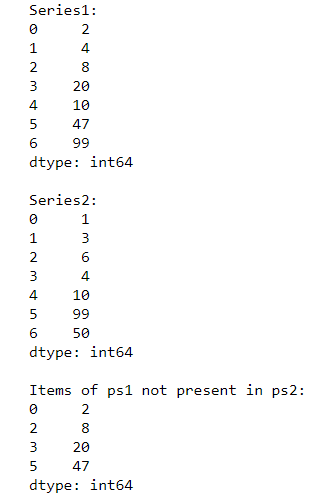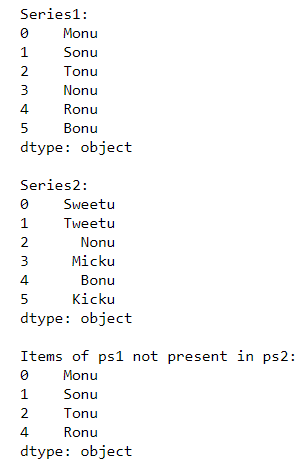# Pandas – Get the elements of series that are not present in other series

• Last Updated : 11 Oct, 2020

Sometimes we have two or more series and we have to find all those elements that are present in one series but not in other. We can do this easily, using the Bitwise NOT operator along with the pandas.isin() function.

Example 1: Taking two Integer Series

## Python3

 `# Importing pandas library ` `import` `pandas as pd ` ` `  `# Creating 2 pandas Series ` `ps1 ``=` `pd.Series([``2``, ``4``, ``8``, ``20``, ``10``, ``47``, ``99``]) ` `ps2 ``=` `pd.Series([``1``, ``3``, ``6``, ``4``, ``10``, ``99``, ``50``]) ` ` `  `print``(``"Series1:"``) ` `print``(ps1) ` `print``(``"\nSeries2:"``) ` `print``(ps2) ` ` `  `# Using Bitwise NOT operator along ` `# with pandas.isin() ` `print``(``"\nItems of ps1 not present in ps2:"``) ` `res ``=` `ps1[~ps1.isin(ps2)] ` `print``(res) `

Output:In the above example, we take 2 pandas series of int type ‘ps1‘ and ‘ps2‘ and find all those elements of ps1 that are not present in ps2.

Example 2: Taking two Floating point Series

## Python3

 `# Importing pandas library ` `import` `pandas as pd ` ` `  `# Creating 2 pandas Series  ` `ps1 ``=` `pd.Series([``2.8``, ``4.5``, ``8.0``, ``2.2``, ``10.1``, ``4.7``, ``9.9``]) ` `ps2 ``=` `pd.Series([``1.4``, ``2.8``, ``4.7``, ``4.8``, ``10.1``, ``9.9``, ``50.12``]) ` ` `  `print``(``"Series1:"``) ` `print``(ps1) ` `print``(``"\nSeries2:"``) ` `print``(ps2) ` ` `  `# Using Bitwise NOT operator along  ` `# with pandas.isin() ` `print``(``"\nItems of ps1 not present in ps2:"``) ` `res ``=` `ps1[~ps1.isin(ps2)] ` `print``(res)`

Output:In the above example, we take 2 pandas series of float type ‘ps1‘ and ‘ps2‘ and find all those elements of ps1 that are not present in ps2.

Example 3: Taking two String Series

## Python3

 `# Importing pandas library ` `import` `pandas as pd ` ` `  `# Creating 2 pandas Series ` `ps1 ``=` `pd.Series([``'Monu'``, ``'Sonu'``, ``'Tonu'``, ``'Nonu'``, ` `                 ``'Ronu'``, ``'Bonu'``]) ` ` `  `ps2 ``=` `pd.Series([``'Sweetu'``, ``'Tweetu'``, ``'Nonu'``, ` `                 ``'Micku'``, ``'Bonu'``, ``'Kicku'``]) ` ` `  `print``(``"Series1:"``) ` `print``(ps1) ` `print``(``"\nSeries2:"``) ` `print``(ps2) ` ` `  `# Using Bitwise NOT operator along with ` `# pandas.isin() ` `print``(``"\nItems of ps1 not present in ps2:"``) ` `res ``=` `ps1[~ps1.isin(ps2)] ` `print``(res) `

Output:In the above example, we take 2 pandas series of string type ‘ps1‘ and ‘ps2‘ and find all those elements of ps1 that are not present in ps2.

My Personal Notes arrow_drop_up
Recommended Articles
Page :